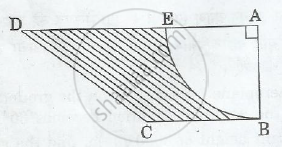# In Figure 2, ABCD is a trapezium of area 24.5 sq. cm. In it, AD|| BC, ∠ DAB = 900, AD = 10 cm and BC = 4 cm. If ABE is a quadrant of a circle, find the area of the shaded region. [Take π=22/7] - Mathematics

In Figure 2, ABCD is a trapezium of area 24.5 sq. cm. In it, AD|| BC, ∠ DAB = 900, AD = 10 cm and BC = 4 cm. If ABE is a quadrant of a circle, find the area of the shaded region. [Take π=22/7]#### SolutionGiven :-
BC = 4 cm
Area of trapezium = 24.5 cm2

Area of trapezium = (a+b)/2 height [

=(AD+BC)/2 AB

24.5=(10+4)/2 AB

24.5/7=AB

AB = 3.5 cm
Area of quadrant = Area of a circle / 4

Area of quadrant = 1/4 xx pi xx r2 = 0.25 xx 22/7 xx3.5 xx 3.5 = 9.625 cm^2

The area of shaded region = Area of trapezium – Area of the given quadrant
The area of shaded region = 24.5 - 9.625 = 14.875 cm2

Concept: Surface Area of a Combination of Solids
Is there an error in this question or solution?